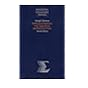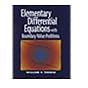Normal view MARC view ISBD view

# Elementary differential equations with boundary value problems / William F. Trench.

Material type:TextPublisher: Pacific Grove, CA : Brooks/Cole-Thomson Learning, c2001Description: xv, 736 p. : ill. ; 25 cm.ISBN: 0534263283; 9780534263287.DDC classification: 515.35 Online resources: WorldCat details
Contents:
Table of contents Introduction -- First order equations -- Numerical methods -- Applications of first order equations -- Linear second order equations -- Applications of linear second order equations -- Series solutions of linear second order equations -- Laplace transforms -- Linear higher order equations -- Linear systems of differential equations -- Boundary value problems and Fourier expansions -- Fourier solutions of partial differential equations -- Boundary value problems for second order ordinary differential equations.
Tags from this library: No tags from this library for this title.Average rating: 0.0 (0 votes)
Item type Current location Collection Call number Copy number Status Date due Barcode Item holdsText
Reserve Section
Non-fiction 515.35 TRE 2001 (Browse shelf) C-1 Not For Loan 12767Text
Circulation Section
Non-fiction 515.35 TRE 2001 (Browse shelf) C-2 Available 12768Text
Circulation Section
Non-fiction 515.35 TRE 2001 (Browse shelf) C-3 Available 12769Text
Circulation Section
Non-fiction 515.35 TRE 2001 (Browse shelf) C-4 Available 12770Text
Circulation Section
Non-fiction 515.35 TRE 2001 (Browse shelf) C-5 Available 12771
Total holds: 0
##### Browsing EWU Library Shelves , Shelving location: Reserve Section Close shelf browser515.35 SID 1991 Differential equations, with applications and historical notes / 515.35 SID 1991 Differential equations, with applications and historical notes / 515.35 SID 1991 Differential equations, with applications and historical notes / 515.35 TRE 2001 Elementary differential equations with boundary value problems / 515.35 ZIF 2001 A first course in differential equations with modeling applications / 515.35 ZIF 2005 A first course in differential equations with modeling applications / 515.35 ZIF 2005 A first course in differential equations with modeling applications /

Online version:
Trench, William F., 1931-
Elementary differential equations with boundary value problems.
Pacific Grove, CA : Brooks/Cole-Thomson Learning, 2001
(OCoLC)680499622

Includes index.

First order equations --
Numerical methods --
Applications of first order equations --
Linear second order equations --
Applications of linear second order equations --
Series solutions of linear second order equations --
Laplace transforms --
Linear higher order equations --
Linear systems of differential equations --
Boundary value problems and Fourier expansions --
Fourier solutions of partial differential equations --
Boundary value problems for second order ordinary differential equations.

Computer Science & Engineering

There are no comments for this item.